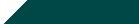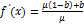``Home| Journals | Statistics Online Expert | About Us | Contact UsUntitled Document

Some Aspects of Dynamical Behavior in a One Dimensional Non Linear Chaotic Map

T. K. Dutta1, A. Kr. Jain2, D. Bhattacharjee3

1Professor, Department of Mathematics, Gauhati University, Guwahati ,Assam, Pin code- 781014, INDIA.

2Assistant Professor, Department of Mathematics, Barama College,Barama, Assam, Pincode-781346,INDIA.

3Assistant Professor, Department of Mathematics, B. Borooah College, Guwahati, Assam, Pin code-781007, INDIA.

Research Article

Abstract: In this paper we consider  an inverse  non linear algebraic ecological model with μ as control parameter and a,b are taken as constants. Here we create a suitable C-programming and use Mathematica software to study period doubling route to chaos. Also to find out an universal route from order to chaos through period doubling bifurcations we set up   proper numerical methods to get periodic points and bifurcation points of different period 2n, where n=0,1,2,3,4,5…..and we successively achieve Feigenbaum Universal  Constant(δ)=4.66920161029…  with the help of bifurcation points calculated numerically. It is also seen that chaotic region takes place beyond accumulation point. We also confirm about chaotic region by getting positive Lyapunov Exponent at some parametric values.

Keywords: Period –Doubling Bifurcation, Periodic points, Feigenbaum Universal Constant, Time Series, Lyapunov Exponent.

1.Introduction

First-order difference equations, although simple and deterministic, can possess an extraordinarily rich spectrum of dynamical behaviour, from stable points, to a bifurcating hierarchy of stable cycles, to apparently random fluctuations.May and Oster stated about the model in their paper in table 1,which is taken from biological literature[8,9,10,13,14] .Stone and Hart(1999) showed  effect of immigration by adding a constant “c” on the model.In this paper we pay our attention to study period doubling route to chaos of an inverse non linear algebraic one dimensional model. We consider our model as   where a, b are constants and μ is the control parameter. Here we first provide the Feigenbaum tree of bifurcation points along with one of the periodic points, which leads to chaos. Secondly, we determine the accumulation point and draw the bifurcation graph of the model and verify that chaos occur beyond accumulation point. Thirdly the graphs of the time series analysis are confirmed in order to support our periodic orbits of period 20 ,21,22,23…..and lastly the graph of Lyapunov exponent confirms   about the existence of the chaotic region.[ 4,5,6]

2. Our vital study

Here the model to be discussed is  .Solving, we get   .At this point we have  ,so maximum value for f(x) is     for μ>0 .We may take the range as[0,   ][ 6]  so as to keep it meaningful to ecological models although the main interest is mathematical.

The solution of gives the fixed points of f(x) .A fixed point x is said to be a (i) stable fixed point or attractor if (ii) unstable fixed point or repeller if1.Solving we get the fixed points as.Now at x=0 ,, so x=0 becomes an unstable point for   . Also at x =  , we getAlso if we consider a= 0.5 ,b =7, then  at x =  , .

Now for  1< μ <1.4 , the absolute value of  remains less than 1,and the point  is stable. As soon as μ>1.4 the point becomes unstable. Hence μ=1.4 is the 1st bifurcation point of this model.

Fig 2.1 Inersection of the model and f(x)=x.Here the abcissa represents x and ordinate represents f.

We next consider the periodic points of period-two and higher.The period-2 points are found by solving the equation   ,where  .Now to solve this equation  analytically is cumbersome one. So we use Newton-Raphson method and bisection method respectively. We build up suitable numerical method and obtain following bifurcation points of different period ,one of the periodic point and Feigenbaum delta(experimental value).

Fig2.2 Graphs of    and

Fig2.3 Graphs of    and

For numerical procedure  first of all I(a,xi*,n) representing the nth  derivative of the function f at  each of the periodic points is calculated numerically and  is calculated. Now the interval [a0,a1] is choosen in such a way that   , where xi* are the periodic points at the parameter a0 and yi* are the periodic points at the parameter a1 for i=1,2,3…,n. Then   is calculated where are the periodic points at the parameter a= and the bisection method process is repeated till the bifurcation point up to certain accuracy is achieved.

The following are some of the bifurcation points obtained with the above process.

Table 2.4:  Calculation  of  bifucation point.

 Bifurcation Point One of the periodic points Feigenbaum delta(experimental value) 1.4000000000000 1.754613 1.575706408457226540 1.445574 1.630043290746602260 1.299966 δ1=3.2336490321873926148761809179173 1.642490397413339580 1.641840 δ2=4.3654227168332621823632156765309 1.645204772722345780 1.653288 δ3=4.5856247827979005265651909953825 1.645788131573193260 1.655620 δ4=4.6530112726718142814428731693413 1.645913168853051720 1.656095 δ5=4.6654794070763076560271607270146 1.656115 δ6=4.6684343229566051115284344588563 1.645945688834907110 1.656113 δ7= 4.6690335015141436067738502435894 1.645946917410687557 1.656118 δ8 = 4.6691660764490105476662516374799 1.645947180534433301 1.656120 δ9 =4.6691938691170565445429865360879 1.652130 δ10=4.66915435884768187804365889581 1.645947248956602490 1.655819 δ11= 4.6691988516575694472925677338974 1.645947251541439730 1.652396 δ12=4.6691942932546112963918513486693

From the above table we can establish the Feigenbaum  up to 4.6692011…..Now the following  bifurcation diagram indicates the universal  route to chaos  for our model .

Fig2.4 (a)Bifurcation graph of the model.The abcissa represents the control parameter and ordinate represents the iterated points.

3. Accumulation point

Using the experimental bifurcation points the sequence of accumulation pointsis calculated with the help of the following formula.

Table3.1: Accumulation points for different values of n

µ∞, 1 =1.6235932315645944017782093800194

µ∞, 2 =1.6458827180086068675824202456858

µ∞, 3 = 1.6458827175932474774970325246657

µ∞, 4 =1.6459445454569357623441342339104

µ∞, 5= 1.6459471193308955094055767204584

µ∞, 6= 1.6459472463699447144735582495296

µ∞, 7= 1.6459472519706112002181189171387

µ∞, 8= 1.6459472522334241941060516283839

µ∞, 9= 1.6459472522453221547696101486903

µ∞, 10= 1.6459472522458772854595187683096

µ∞, 11= 1.6459472522458940791899784228632

µ∞, 12= 1.6459472522459031531614616585986

µ∞, 13= 1.6459472522459083077829755483082

The above sequence converges to the value 1.64594725224590...which is the required accumulation point. 

4. Time Series Analysis: [4,6,15] The key theoretical tool used for quantifying chaotic behavior is the notion of a time-series of data for the system. By observing data over a period of time, one can easily understand what changes have taken place in the past. Such an analysis is extremely helpful in predicting the future dynamical behaviour.[5,15]

We open our journey with a couple of very simple time series experiments. On the horizontal axis, the number of iterations (time) is marked, while on the vertical axis the amplitudes (ranging from 1 to 3 ) are given for each iteration.   Figure 4.1 shows the computed time series of x- values starting at x = 1.4 with the parameter value at μ = 1.35 (which is slightly smaller than   the points are connected by line segments. Time series graph is non-sensitive, stable behaviour and leads to the same final state of a single fixed point.

No of iterations

Fig 4.1: Time series graph for period 1

Now, let us look at the second time series in fig 4.2, which is based on the same formula and the same initial value of x with the parameter value μ = 1.5(which is slightly greater than   We notice periodicity and oscillate between two fixed points with the same amplitude, and the cycle repeats .

No of iterations

Fig 4.2: Time series graph for the period 2

The third time series in fig 4.3, which is based on the same formula and the same initial value of x with the parameter value   μ = 1.637. We notice periodicity and oscillate between four fixed points with the same amplitude, and the cycle repeats.

No of iterations

Fig 4.3:  Time Series graph for the period 4.

The fourth time series in fig 4.4, which is based on the same formula and the same initial value of x with the parameter value   μ = 1.637. We notice periodicity and oscillate between four fixed points with the same amplitude, and the cycle repeats.

No of iterations

Fig 4.4:  Time series graph for the period 8 behaviour

But, if we start with the same initial value of x and the parameter value μ = 1.644, the picture shows an irregular pattern which is difficult to predict meaning thereby the appearance of the chaotic region, Fig 4.5. Thus, the time series analysis also helps us for full description of bifurcations and chaos for the concerned model.

No of iterations

Fig 4.5:  Time series graph for the Chaotic behaviour

5 .Lyapunov Exponent

In order to verify how much accurate is the accumulation point, the lyapunov exponent is calculated. Lyapunov exponent at the parameter greater than the accumulation point is found to be positive whereas lyapunov exponent less than the accumulation point is negative and at the accumulation point it should be equal to zero. We begin by considering an attractor point x0  and calculate the Lyapunov exponent, which is the average of the sum of logarithm of the derivative of the function at the iteration points.

The formula may be summarized as follows:[2,7]

Lyapunov exponent (μ) = (log|f/(x0)|+ log|f/(x1)|+ log|f/(x2)|+ log|f/(x3)|+……+ log|f/(xn)|)

From graph of  Lyapunov exponent ,we see that  some portion lie in the negative side of the parameter axis indicating regular behavior (periodic orbits) and  the portion lie on the positive side of the parameter axis  confirm us about the existence of chaos for our model .

Fig:5.1  Lyapunov exponent of the map.Negative values indicate periodic.Almost zero values indicate bifurcation points and positive values indicate chaos.

References:

1. Arrowsmith.D.K and Place.C.M, "An Introduction to Dynamical Systems "Cambridge University Press, 1994.
2. Devaney.R.L,"An introduction to chaotic dynamical systems"2th ed, Addison Wesley, 1989.
3. Denny.G, "Encounters with Choas"McGraw-Hill, Inc.1992
4. Das. N(2012),Some aspect of Bifurcations And Chaos on Non -linear Population Models , Ph.D Thesis, Department of mathematics ,Gauhati University pp33-69
5. Das.N,and Dutta.N (2010)Time series analysis and Lyapunov exponents for a fifth degree chaotic map,Far  East Journal of Dynamical systems Vol.14,No.2 , pp 125-140.
6. Dutta.T.K, and Bhattacharjee.D, Bifurcation, Lyapunov Exponent and fractal Dimensions in a Non-linear Map Advances In Theoretical And Applied Mathematics,Vol-7,No-3(2012),pp-223-235.
7. Hilborn, R. C., Chaos and Nonlinear Dynamics: An Introduction for Scientists and Engineers, Oxford University Press, 1994.
8. Leslie,P.H.(1957) An analysis of the data for some experiments carried out by Gause with populations of the protozoa,Paramecium aurelia and p.caudatum. Biometrika V-44:pp.314-327.
9. May,R.M(1976) Simple mathematical models with very complicated dynamics ,Nature,261:459-67.
10. May.R.M and Oster,G.F (1976)  Bifurcations and Dynamic Complexity in Simple Ecological Models ,American Naturealist ,pp573-599,V.110,Issue974.
11. Smith,M.J,(1974);Models in ecology.Cambridge University Press,Cambridge.
12. Stone,L.,Hart,D.,Effects of immigration on the Dynamics of Simple population model.
13. Skellam,J.G (1951) Random dispersal in theoretical populations.Biometrika 38:pp 196-218.
14. Utida,S.(1967) Damped oscillation of population density at equilibrium. Res. pop. Ecol. V.9 pp.1-9.
15. Sarmah,H.K and Paul,R. (2012)Chaos in logistic map with emigration. IJTAP V.2  No.1 pp125-144.

Corresponding author:

Anil Kumar Jain, Assistant Professor, Department of Mathematics, Barama College, Barama, Assam, Pin code-781346, INDIA.

Email: [email protected]